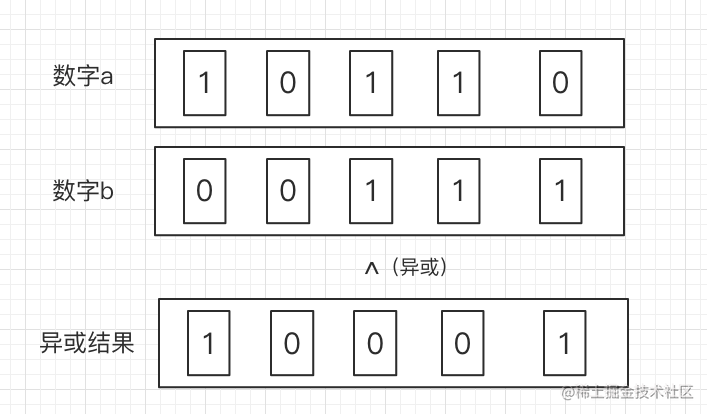# 前端也要会【异或运算】

## 运算规律

### 相同为0，不同为1### 一个值于0异或，都是这个数

``````a ^ 0 = a

### 任何数跟自己异或，都为0

``````a ^ a = 0

### 满足交换律和结合律

``````a ^ b = b ^ a;

``````(a ^ b) ^ c = a ^ (b ^ c)

## 案例

### 两值交换（不借助变量）

``````let a = 5;
let b = 10;
// 值交换
a = a ^ b;
b = a ^ b;
a = a ^ b;

console.log('a=>', a)
console.log('b=>', b)

``````a = a ^ b;  // 代码执行

``````b = a ^ b;  // 代码执行

``````a = a ^ b; // 代码执行

### 简单加解密

``````const cipher = text ^ key;

``````const text = cipher ^ key;

``````const cipher = text ^ key;
const text = (text ^ key) ^ key;

### 算法题

``````const a = [2,3,4,5,6,5,5,6,4,3,2];

// 输出奇数
number = 5;

``````const arr = [2,3,4,5,6,5,5,6,4,3,2];
let number = arr;
for (var i = 1; i < arr.length; i ++) {
number = number ^ arr[i]
}

console.log('number=>', number);

``````a = 2;
b = 3;
c = 4;
d = 5;
e = 6;

// 得到
a ^ b ^ c ^ d ^ e ^ d ^ d ^ e ^ c ^ b ^ a;
// 进一步处理得到
a ^ a ^ b ^ b ^ c ^c ^ d ^ d ^ e ^ e ^ d
// 输出 d

## 结束语

1、点赞，让更多的人也能看到这篇内容（收藏不点赞，都是耍流氓 -_-）

2、关注我，让我们成为长期关系

3、关注公众号「前端有话说」，里面已有多篇原创文章，和开发工具，欢迎各位的关注，第一时间阅读我的文章